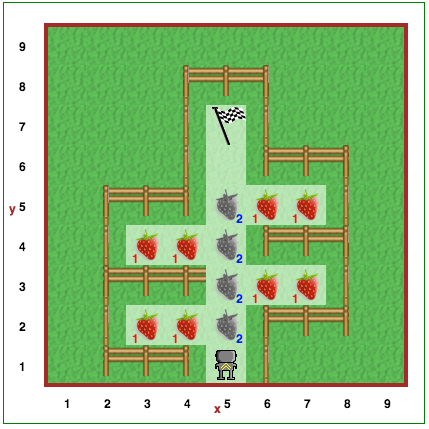# Step 5: Defining New Functions¶

## Tutorial¶

After completing the last step, you have realized that having Reeborg make three left turns in a row gives the same final result as if it were to make a single right turn. It was probably becoming quite tedious to have to write three `turn_left()` instructions each time you want to have Reeborg turn right. Wouldn’t it be nicer if you could simply write `turn_right()`?

Just as we can call built-in functions like `turn_left()` and `move()`, we can create our own functions as well. We can define a new Python function as follows:

```def some_well_chosen_name():
# some lines of code
# indented at the same level
```

`def` is the first Python keyword we have encountered. Keywords are words that have a special meaning in a given programming language. Notice how a colon `:` precedes what is known as a block of code, and the indented portion that follows is the body of the function. It is required in Python to indent such blocks of code with the same number of spaces at the beginning of each line which, as a side-effect, makes it easy to identify the function body for a human reader.

Let’s write our first Python function:

```def turn_right():
turn_left()
turn_left()
turn_left()
```

That’s it! You will now be able to avoid having to write three `turn_left()` functions in a row to simulate a right turn!

### How to think about `def`¶

Note

Please note that this simplified explanation does not take into account what is known as variable scope which is something that we will cover later.

You have just seen how to define a function in Python. Chances are, you understood right away how to think about them but, just in case, here’s a more detailed explanation which will help you to not only understand how functions work, but also other Python construct that involve blocks of code.

Suppose we have the following:

``` def turn_right():  # begin of code block follows
turn_left()
turn_left()
turn_left()   # end of code block

move()
turn_right()
move()
```

This is equivalent to the following:

``` # define a function
def turn_right():
turn_left()
turn_left()
turn_left()

move()
# begin of code block inside turn_right()
turn_left()
turn_left()
turn_left()
# end of code block
move()
```

In other words, `def` defines a name that we can use as a synonym for all the code that appears inside the code block, and whenever we see the synonym being called [that is, the name appears followed by `()`], we can think of it as being equivalent to inserting the code block as is at that location.Reeborg wants to pick some strawberries in its garden. Reeborg’s garden has a very strange shape, as you’ve seen above. Define a `pick_two_berries()` function that picks up two strawberries and puts them on the ground. Use it four times as part of your solution. As always, be sure to use comments and whitespace to increase the readability of your solution!